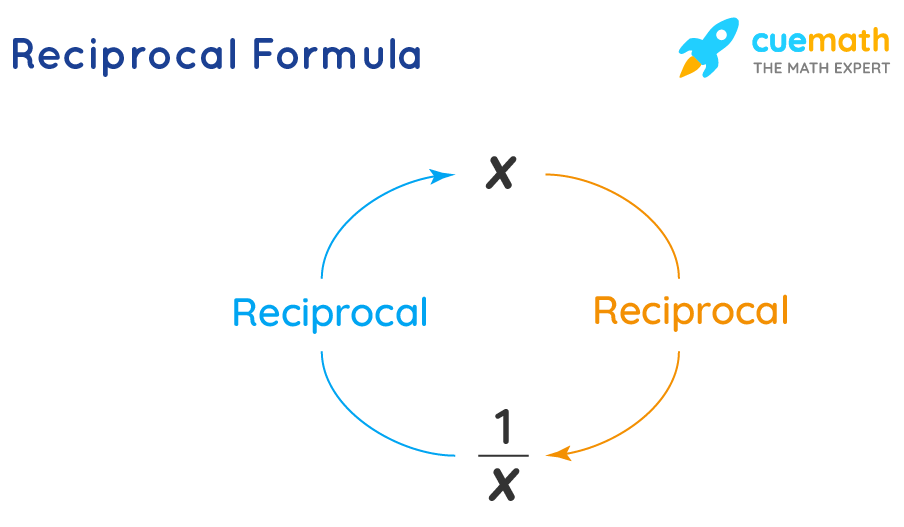# Reciprocal Formula

Go back to  'Math Formulas'

Reciprocal means an inverse of a number or value. If x is any real number, then the reciprocal of this number will be 1/x. For example, the reciprocal of 8 is 1 divided by 8, i.e. 1/8.
Also, if we multiply a number by its reciprocal, it gives one (1) as a result.

Let us learn about the reciprocal formula with a few examples in the end.

## What Is Reciprocal Formula?

The Reciprocal Formula of a given number can be expressed as,

$$\dfrac{1}{x} \,\,\text{or}\,\, {x^-1}$$## Solved Examples Using Reciprocal Formula.

### Example 1:

What number would give the product as 1 when multiplied by14?

Solution:

To find: We need to determine the reciprocal of 14

14 is a natural number. (given)

Using Reciprocal Formula,

the reciprocal of 14 = $$\dfrac{1}{14}$$

$$\dfrac{1}{14}$$ on multiplying with 14 gives 1:

$$\dfrac{1}{14} \times 14 = 1$$

Answer: $$\dfrac{1}{14}$$ when multiplied by 14 gives 1.

### Determine the reciprocal of $$1\dfrac{1}{2}$$.

Solution:

Firstly, let's convert the mixed fraction into an improper fraction.

$$1\dfrac12 = \dfrac32$$

To find: The reciprocal of $$\dfrac{3}{2}$$

Using Reciprocal Formula,

Reciprocal of $$\dfrac{3}{2}$$ is $$\dfrac{2}{3}$$

Answer: $$\dfrac{2}{3}$$ is the reciprocal of $$\dfrac{3}{2}$$.